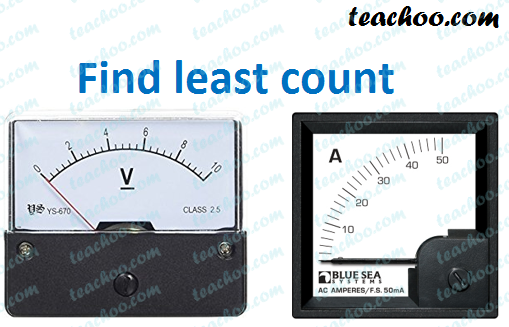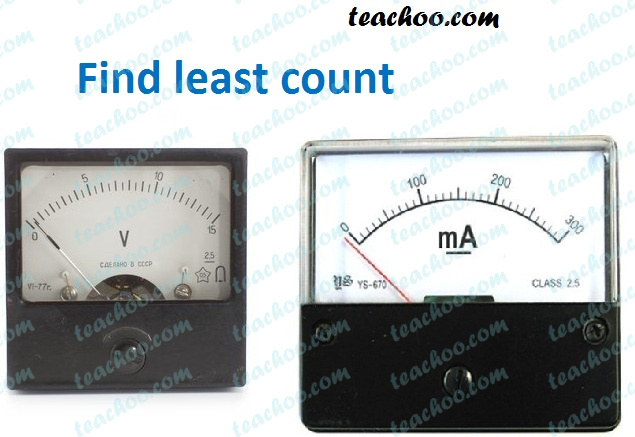Teachoo Questions

Class 10
Chapter 12 Class 10 - Electricity

The smallest value that can be measured is called Least Count .

In Ammeter and Voltmeter, the smallest value that can be measured will be least count.

Let's do this by some examples

## Find Least count of Ammeter and Voltmeter given belowHere,

 Voltmeter Ammeter For Voltmeter,  We see that 5 Division is equal to 2 Volts   So,  Least Count = 2/5 = 0.4 V For Ammeter,  We see that 5 Division is equal to 10 Amperes   So,  Least Count = 10/5 = 2 A

So, Least Count of Voltmeter is 0.4 V and Least count for Ammeter is 2A

## Find Least count of Ammeter and Voltmeter given belowVoltmeter Ammeter For Voltmeter,  We see that 10 Divisions is equal to 5 Volts   So,  Least Count = 5/10 = 0.5 V For Ammeter,  We see that 10 Divisions is equal to 100 Milli Amperes   So,  Least Count = 100/10 mA = 10 mA = 10 × 10 -3 A = 10 -2 A = 0.01 A

So, Least Count of Voltmeter is 0.5 V and Least count for Ammeter is 0.01 A

## Find Least count of Ammeter and Voltmeter given belowAmmeter Voltmeter For Ammeter,  We see that 10 Divisions is equal to 0.2 Amperes   So,  Least Count = 0.2/10 = 0.02 A For Voltmeter,  We see that 10 Division is equal to 1 Volt   So,  Least Count = 1/10 = 0.1 V

So, Least Count of Voltmeter is 0.4 V and Least count for Ammeter is 0.02 A

## An ammeter has a range of 0-3 ampere and there are 30 divisions on its scales. What is its least count?

This means that

In Ammeter

30 Division is 3 A

So,

Least Count = 3/30

= 1/10

= 0.1 A

So, Least Count of Ammeter is 0.1 A

Get live Maths 1-on-1 Classs - Class 6 to 12